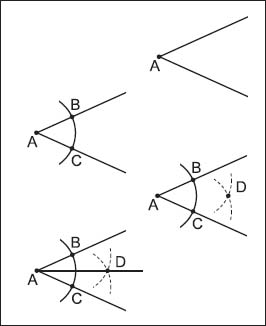NextPrevious

# What are geometric postulates?

Similar to other parts of mathematics, there are many geometric postulates, or statements that are assumed to be true without proof. From these postulates, theorems—another type of mathematical statement—can be proven (in addition, theorems are proven by definitions or previously proven theorems). An example of a postulate in geometry is, “Through any two points there is exactly one line.” Another is, “If two points lie in a plane, then the entire line containing those two points lies in the plane.”To determine a bisector, follow these steps from top to bottom: Draw an angle; draw an arc from the angle’s vertex that touches the two angle lines; draw two more arcs from the points where the first arc meets the angle lines; draw a line from point A to point D.

Close

This is a web preview of the "The Handy Math Answer Book" app. Many features only work on your mobile device. If you like what you see, we hope you will consider buying. Get the App